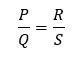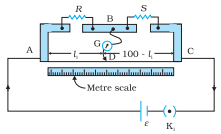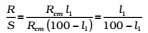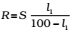# Meter Bridge or slide Wire Bridge

0
209

## What Is Wheatstone Bridge?

A Wheatstone bridge is a kind of electrical circuit used in measuring an electrical resistance, which is unknown by balancing its two legs of the bridge circuit, where one of the legs includes an unknown component. Samuel Hunter Christie created this instrument in the year 1833 and was improved and also simplified by Sir Charles Wheatstone in the year 1843. The digital multimeters in today’s world provide the simplest forms in measuring the resistance. The Wheatstone Bridge can still be used in measuring light values of resistances around the range of milli-Ohms.

It is the simplest practical application of the Wheatstone bridge that is used to measure an unknown resistance.

### Principle:

Its working is based on the principle of Wheatstone bridge.

When the bridge is balanced,Fig. A meter bridge. Wire AC is 1 m long. R is a resistance to be measured and S is a standard resistance.

### Construction:

It consists of a wire of length 1m and of uniform cross-sectional area stretched taut and clamped between two thick metallic strips bent at right angles, as shown.

The metallic strip has two gaps across which resistors can be connected. The endpoints where the wire is clamped are connected to a cell through a key.

One end of a galvanometer is connected to the metallic strip midway between the two gaps. The other end of the galvanometer is connected to a ‘jockey’.

The jockey is essentially a metallic rod whose one end has a knife-edge which can slide over the wire to make an electrical connection.

### Working:

The jockey can be moved along the wire. The portion AD of the wire has a resistance Rcml, where Rcm is the resistance of the wire per unit centimeter. The portion DC of the wire similarly has a resistance Rcm (100-l).

The four arms AB, BC, DA and CD [with resistances RSRcmand Rcm(100-l)] obviously form a Wheatstone bridge with AC as the battery arm and BD the galvanometer arm. If the jockey is moved along the wire, then there will be one position where the galvanometer will show no current.Thus, once we have found out l1, the unknown resistance is known in terms of the standard known resistance byKnowing l and R, unknown resistance S can be determined.

### Procedure For Finding The Unknown Resistance Using Meter Bridge

• Collect the instruments and prepare connections as shown in the above figure.
• Take some suitable kind of resistance ‘R’ from the resistance box.
• Touch jockey at the point A; look that there exists a deflection in galvanometer on one of the sides, then contact the jockey on point C of wire, then the deflection in galvanometer has to be on another side.
• Find the position of the null point having deflection in the galvanometer that becomes zero. Note the length AB (l) BC = (100 – l).
• Continue the above method for some different values of the ‘R’. Note at least some 5 readings.
• Consider the point where galvanometer shows a 0 deflection; this is called balance point.
• Now, Measure the length of given wire by the use of ordinary scale and radius of the wire by the utilization of a screw gauge, (Take at least five readings).
• Calculate Mean Resistance of Single Unknown Resistance = Total Sum of resistances of Unknown resistance from the above five readings)/5.